Ex 7.1

Chapter 7 Class 10 Coordinate Geometry
Serial order wise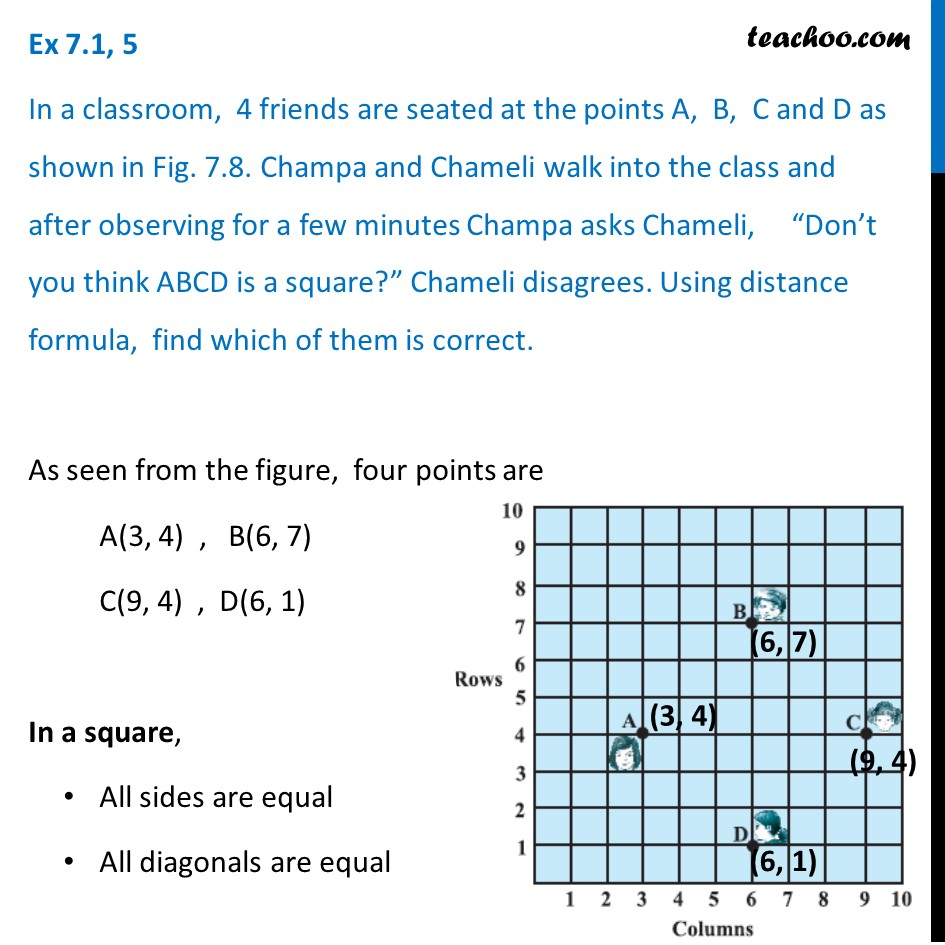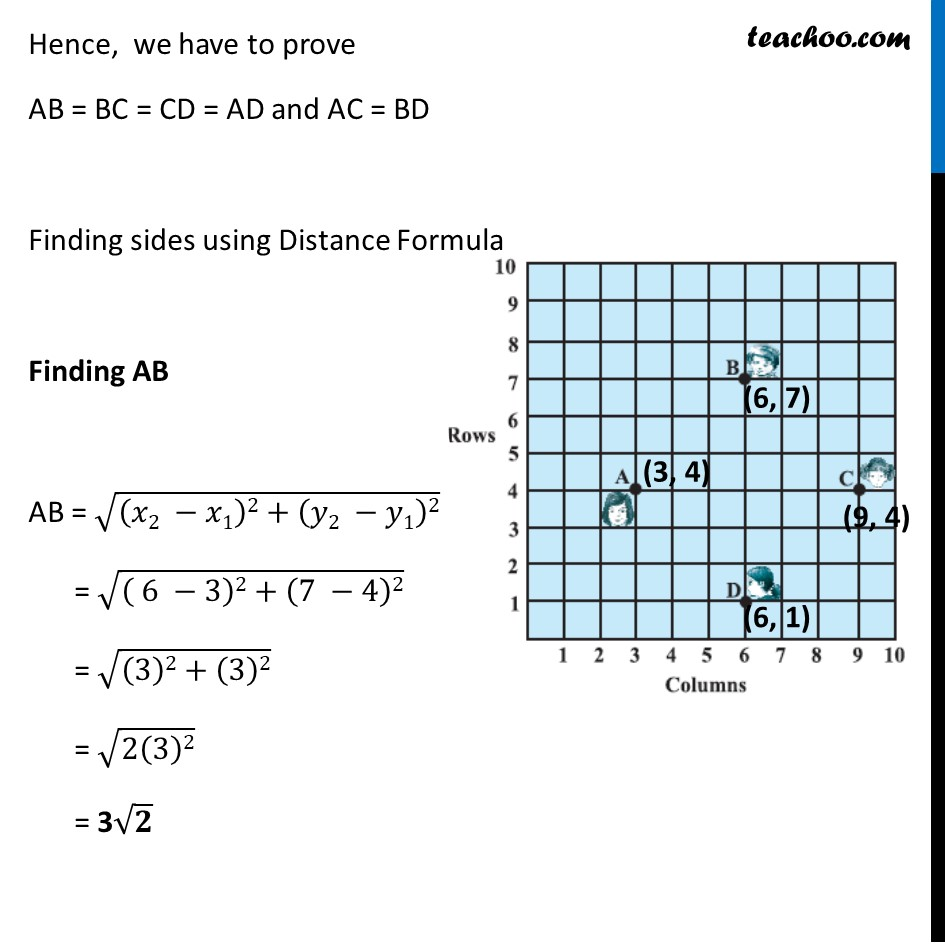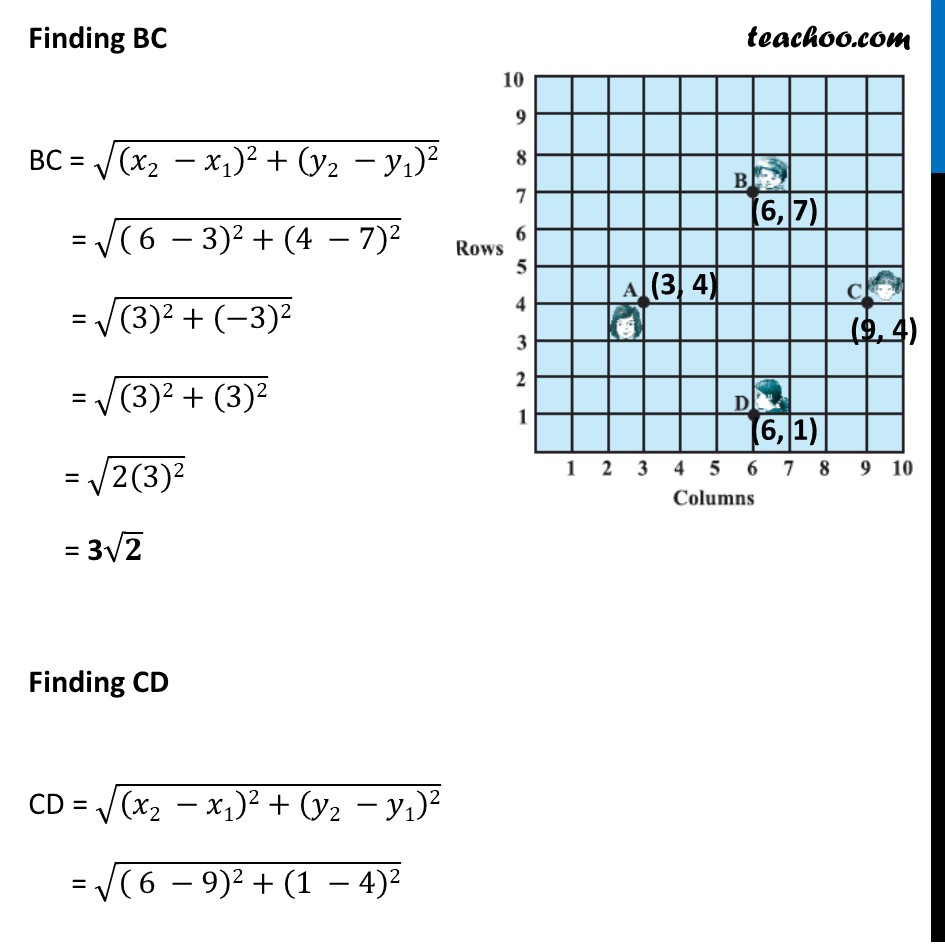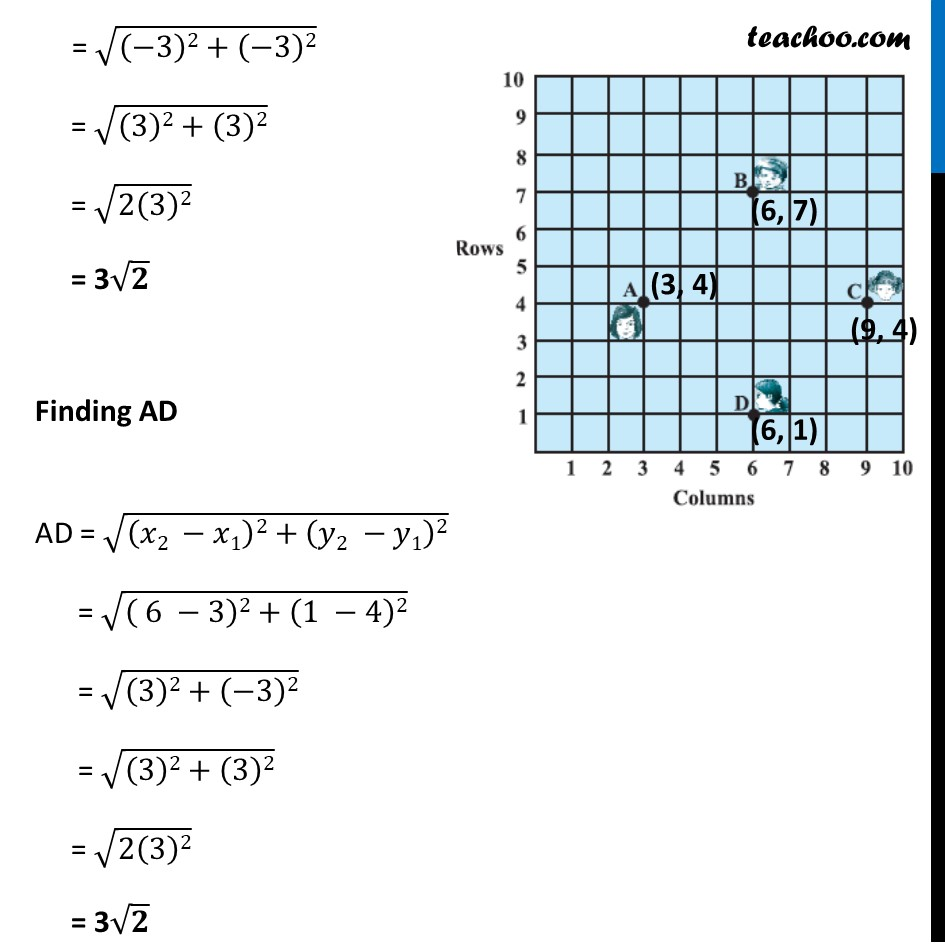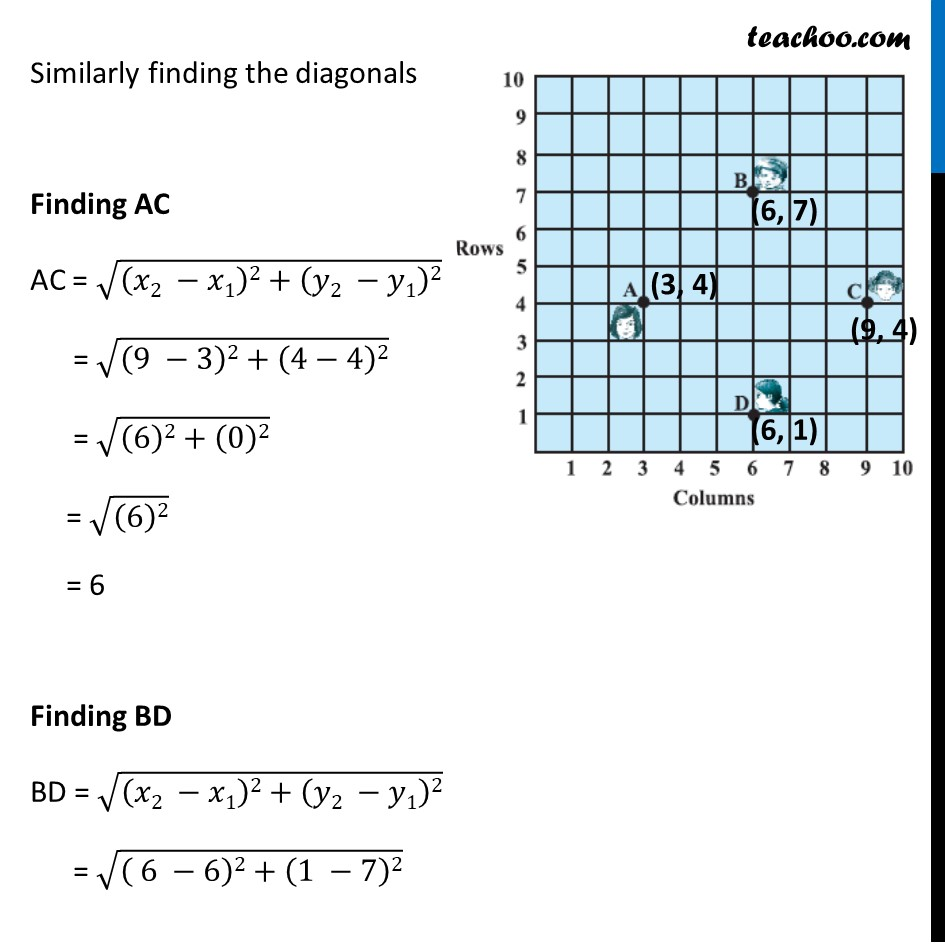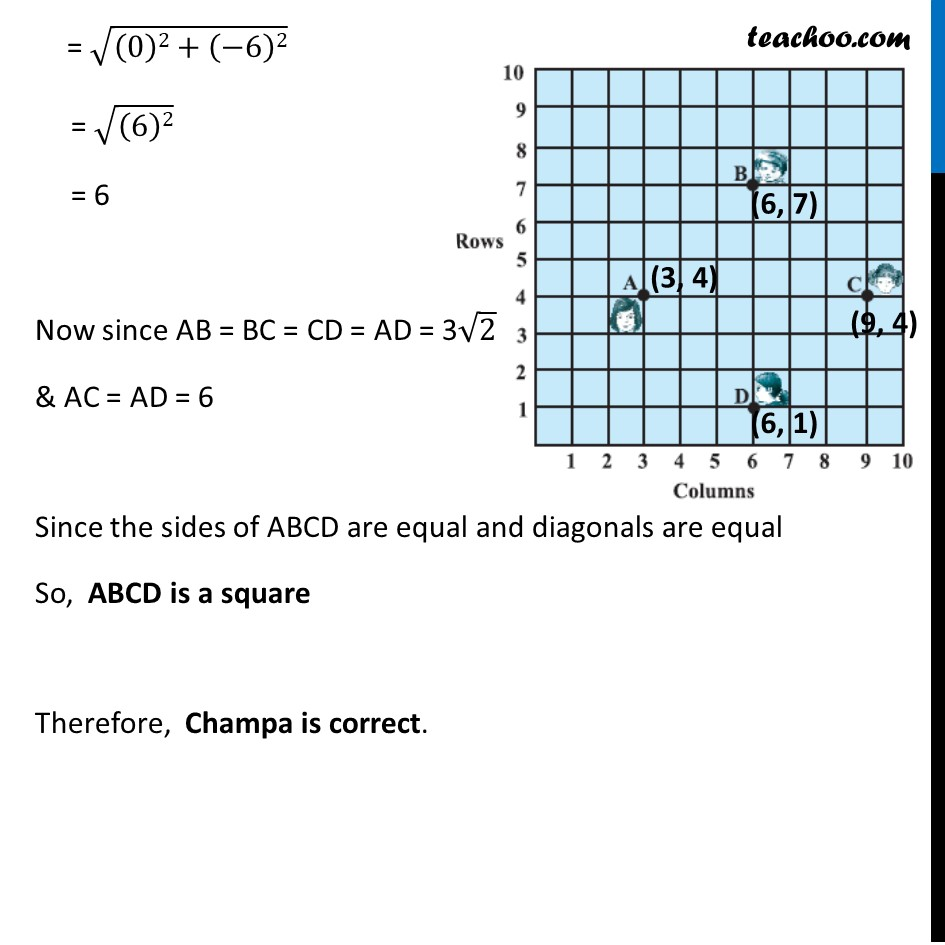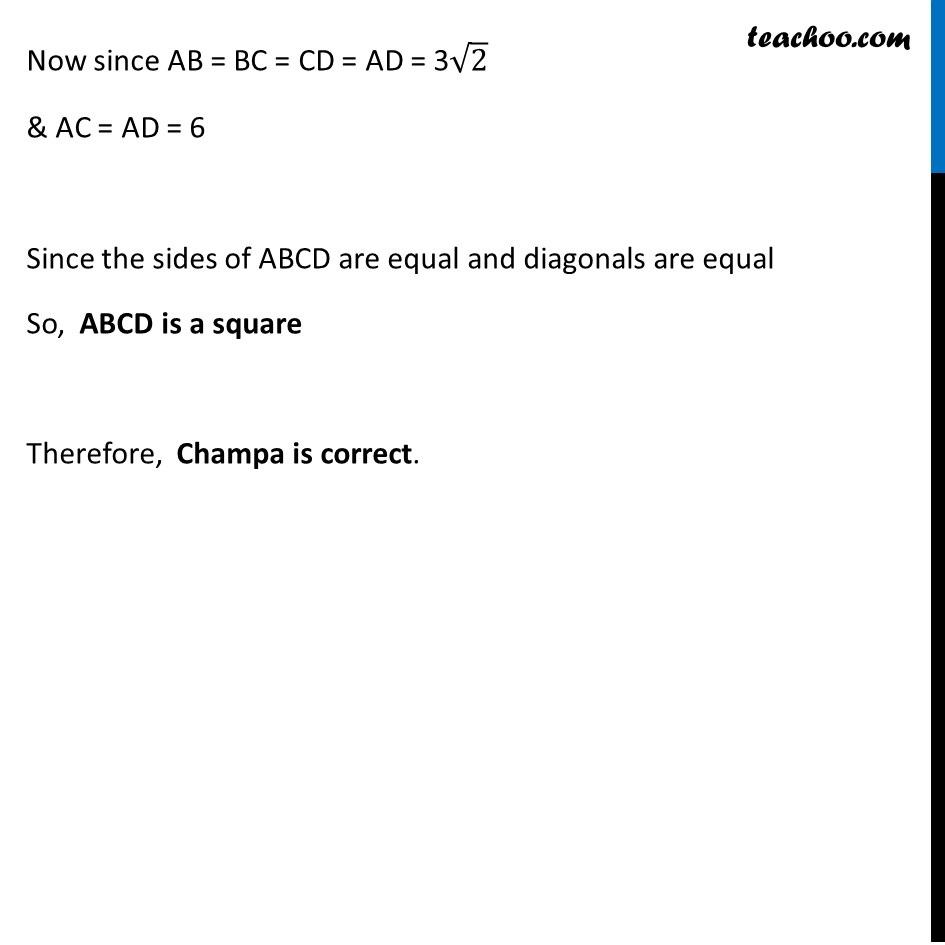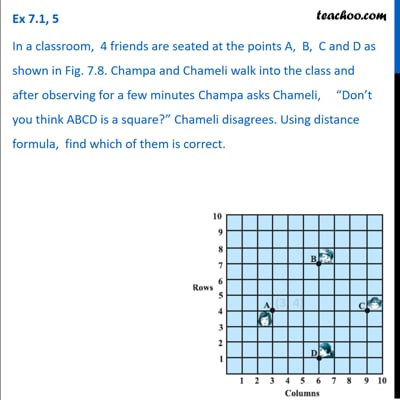This video is only available for Teachoo black users

Learn in your speed, with individual attention - Teachoo Maths 1-on-1 Class

### Transcript

Ex 7.1, 5 In a classroom, 4 friends are seated at the points A, B, C and D as shown in Fig. 7.8. Champa and Chameli walk into the class and after observing for a few minutes Champa asks Chameli, “Don’t you think ABCD is a square?” Chameli disagrees. Using distance formula, find which of them is correct. As seen from the figure, four points are A(3, 4) , B(6, 7) C(9, 4) , D(6, 1) In a square, All sides are equal All diagonals are equal Hence, we have to prove AB = BC = CD = AD and AC = BD Finding sides using Distance Formula Finding AB AB = √((𝑥2 −𝑥1)2+(𝑦2 −𝑦1)2) = √(( 6 −3)2+(7 −4)2) = √((3)2+(3)2) = √(2(3)2) = 3√𝟐 Finding BC BC = √((𝑥2 −𝑥1)2+(𝑦2 −𝑦1)2) = √(( 6 −3)2+(4 −7)2) = √((3)2+(−3)2) = √((3)2+(3)2) = √(2(3)2) = 3√𝟐 Finding CD CD = √((𝑥2 −𝑥1)2+(𝑦2 −𝑦1)2) = √(( 6 −9)2+(1 −4)2) = √((−3)2+(−3)2) = √((3)2+(3)2) = √(2(3)2) = 3√𝟐 Finding AD AD = √((𝑥2 −𝑥1)2+(𝑦2 −𝑦1)2) = √(( 6 −3)2+(1 −4)2) = √((3)2+(−3)2) = √((3)2+(3)2) = √(2(3)2) = 3√𝟐 Similarly finding the diagonals Finding AC AC = √((𝑥2 −𝑥1)2+(𝑦2 −𝑦1)2) = √((9 −3)2+(4−4)2) = √((6)2+(0)2) = √((6)2) = 6 Finding BD BD = √((𝑥2 −𝑥1)2+(𝑦2 −𝑦1)2) = √(( 6 −6)2+(1 −7)2) = √((0)2+(−6)2) = √((6)2) = 6 Now since AB = BC = CD = AD = 3√2 & AC = AD = 6 Since the sides of ABCD are equal and diagonals are equal So, ABCD is a square Therefore, Champa is correct. Now since AB = BC = CD = AD = 3√2 & AC = AD = 6 Since the sides of ABCD are equal and diagonals are equal So, ABCD is a square Therefore, Champa is correct.